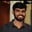Related Tags

communitycreator
c

# How to transpose a square matrix in CBuchiredddypalli Koushik

### Overview

Read one matrix (called Matrix 1) using the standard input (scanf), and try to transpose the matrix to get the result.

Transpose matrix means the original matrix rows will be changed to columns and columns to rows.

The result is stored in the new matrix, which is the transpose matrix.

### Example

Let’s first take a 2x2 matrix and try to transpose it.

Matrix 1:

$\begin{bmatrix} 1 & 2 \\ 3 & 4\\ \end{bmatrix} \quad$

The resultant matrix will look like this :

Transpose matrix:

$\begin{bmatrix} 1 & 3 \\ 2 & 4\\ \end{bmatrix} \quad$

### Algorithm

Generally, the transpose of the matrix is denoted by T.

When reading the data, we need to use loops.

We also need loops to access the transpose of the matrix.

After transposing the elements, we store the data in the resultant matrix.

The pseudo code looks like this:

for(i=0;i<N; i++)
{
for(j=0;j<N ;j++)
{
Transpose[j][i] = Matrix[i][j];
}
}

This is the original matrix
1 of 2

### Code

To run the code below, first give the input value of n (size), followed by values of the matrix.

After giving all the inputs, we’ll get the resultant matrix which is the transpose of Matrix 1.

Note: For a 2x2 matrix, we can give the values of a matrix as follows: 2 1 2 3 4

Here, 2 is the value of n and we have 4 values for the matrix. Here, “2 1 2 3 4” would be the input.

#include<stdio.h>
int main()
{
int i, j,  N; // Declaration of variables.
scanf("%d", &N); // Reading the value of n which represnts size of the matrix.
int Matrix[N][N], Transpose[N][N]; // Declaring the matrices.
for(i=0;i<N;i++)
{
for(j=0;j<N;j++)
{
scanf("%d",&Matrix[i][j]);
}
}
// Transposing the matrix
for(i=0;i<N;i++)
{
for(j=0;j<N;j++)
{
Transpose[j][i] =Matrix[i][j]; // In this step we interchange the rows ans columns.
}
}
// Printing the matrix
for(i=0;i<N;i++)
{
for(j=0;j<N;j++)
{
printf("%d ",Transpose[i][j]);
}
printf("\n");
}

return 0;
}

Enter the input below

Transpose of a matrix

### Explanation

• Line 4: The N represents the dimensions of the matrix.

• Line 6: We declare two matrices. The matrix Matrix[n][n] represents the original matrix and the matrix Transpose[n][n] represents the transpose of the original matrix.

• First, we take the value of N, which represents the size of the matrix.
• Line 8: We read the first matrix using the for loop.

• Line 16: After reading the matrix, we transpose the matrix. In this step, we interchange the rows with columns or columns with rows.

• Line 24: This transposition of the matrix is put into the resultant matrix, and finally, we use the for loop to print the final resultant matrix.

RELATED TAGS

communitycreator
c

CONTRIBUTORBuchiredddypalli Koushik
RELATED COURSES

View all Courses

Keep Exploring

Learn in-demand tech skills in half the time